#Nowadays, artificial intelligence is present in almost every part of our lives. Smartphones, social media feeds, recommendation engines, online ad networks, and navigation tools are some examples of AI-based applications that already affect us every day. Deep learning in areas such as speech recognition, autonomous driving, machine translation, and visual object recognition has been systematically improving the state of the art for a while now.

However, the reasons that make deep neural networks (DNN) so powerful are only heuristically understood, i.e. we know only from experience that we can achieve excellent results by using large datasets and following specific training protocols. Recently, one possible explanation was proposed, based on a remarkable analogy between a physics-based conceptual framework called renormalization group (RG) and a type of neural network known as a restricted Boltzmann machine (RBM).

#### RG and RBMs as Coarse-Graining Processes

Renormalization is a technique used to investigate the behavior of physical systems when information about its microscopic parts is unavailable. It is a “coarse-graining” method which shows how physical laws change as we zoom out and examine objects at different length scales, “putting on blurry glasses”.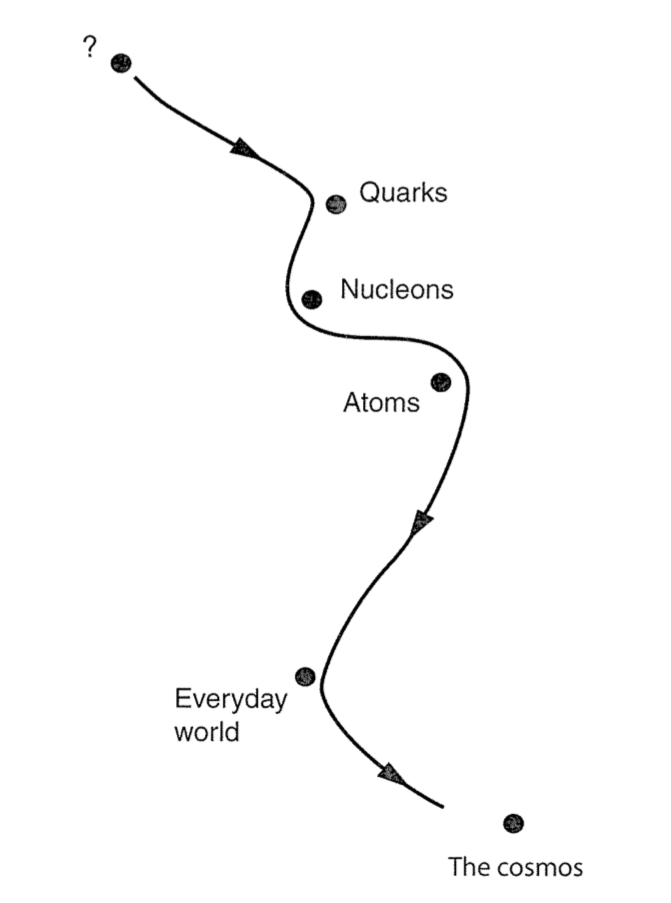When we change the length scale with which we observe a physical system (when we “zoom in”), our theories “navigate the space” of all possible theories (source).

The great importance of the RG theory comes from the fact that it provides a robust framework that essentially explains why physics itself is possible.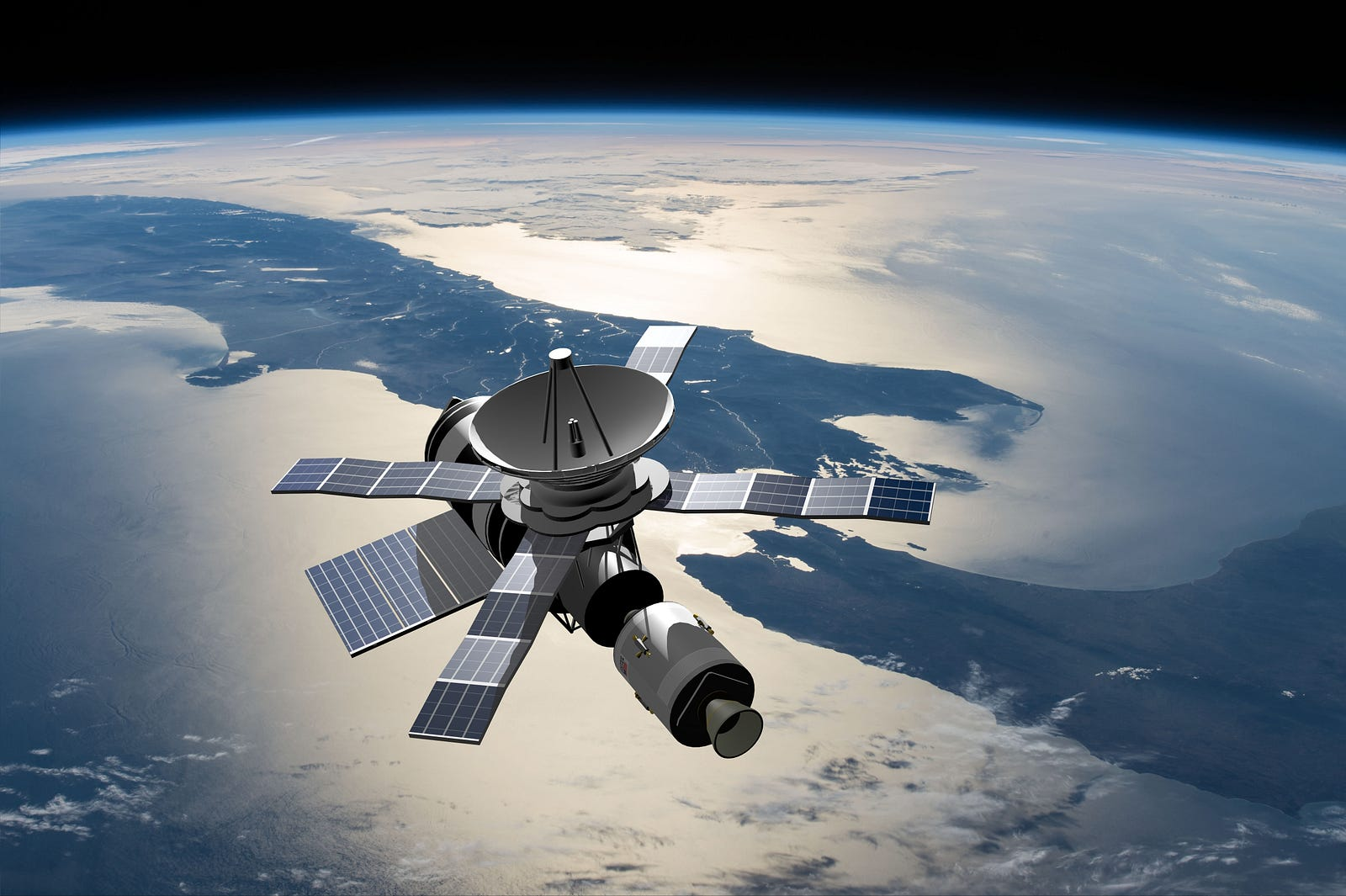To describe the motion of complex structures such as satellites, one does not need to take into account the motions of all its constituents. Picture by 3Dsculptor/Shutterstock.com.

RG theory provides a robust framework that explains why physics itself is possible.

For example, to compute the trajectory of a satellite orbiting the Earth we merely need to apply Newton’s laws of motion. We don’t need to take into account the overwhelmingly complex behavior of the satellite’s microscopic constituents to explain its motion. What we do in practice is a sort of “averaging” of the detailed behavior of the fundamental components of the system (in this case the satellite). RG theory explains why this procedure works so remarkably well.

Furthermore, RG theory seems to suggest that all our current theories of the physical world are just approximations to some yet unknown “true theory” (in more technical terms, this true theory “lives” in the neighborhood of what physicists call fixed points of the scale transformations).

RG theory seems to suggest that all our current theories of the physical world are just approximations to some yet unknown “true theory”.

RG works well when the system under investigation is at a critical point and displays self-similarity. A self-similar system is “exactly or approximately similar to a part of itself” in whatever length scale it is being observed. Examples of systems displaying self-similarity are fractals.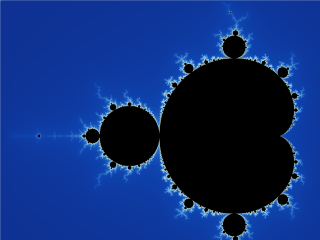Wikipedia animation showing the Mandelbrot set and we zoom in (source).

Systems at critical points display strong correlations between parts of it that are extremely far apart from each other. All subparts influence the whole system and the physical properties of the system become fully independent of its microscopic structure.

Artificial neural networks can also be viewed as a coarse-graining iterative process. ANNs are composed of several layers, and as illustrated below, earlier layers learn only lower-level features from the input data (such as edges and colors) while deeper layers combine these lower-level features (fed by the earlier ones) into higher-level ones. In the words of Geoffrey Hinton, one of the leading figures in the deep learning community: “You first learn simple features and then based on those you learn more complicated features, and it goes in stages.” Furthermore, as in the case of the RG process, deeper layers keep only features that are considered relevant, deemphasizing irre....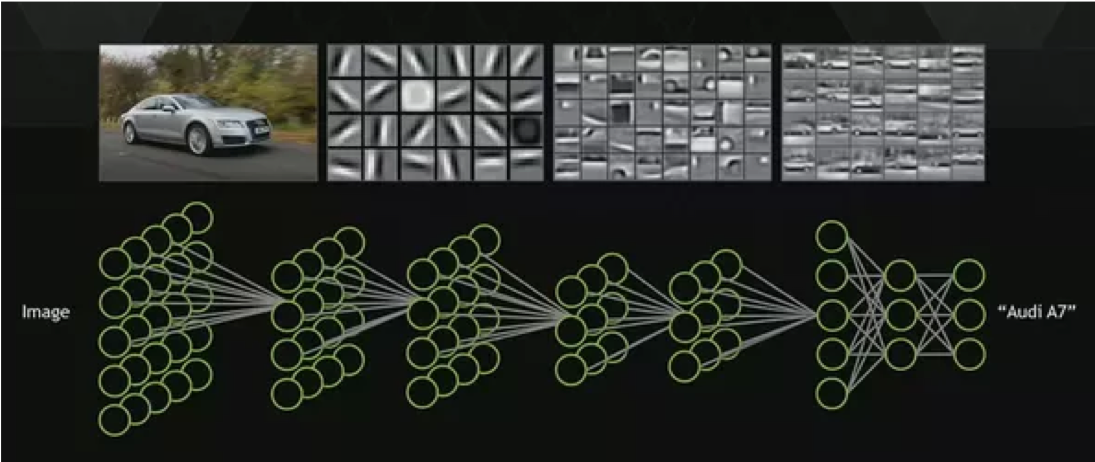Convolutional neural network (CNN). The complexity level of the forms recognized by the CNN is higher in later layers (source).

### An Exact Connection

Both physics and machine learning deal with systems with many constituents. Physics investigates systems containing many (interacting) bodies. Machine learning studies complex data comprising a large number of dimensions. Furthermore, similarly to RG in physics, neural networks manage to categorize data such as, e.g. pictures of animals regardless of their component parts (such as size and color).

In an article published in 2014, two physicists, Pankaj Mehta and David Schwab, provided an explanation for the performance of deep learning based on renormalization group theory. They showed that DNNs are such powerful feature extractors because they can effectively “mimic” the process of coarse-graining that characterizes the RG process. In their words “DNN architectures […] can be viewed as an iterative coarse-graining scheme, where each new high-level layer of the NN learns increasingly abstract higher-level features from the data”. In fact, in their paper, they manage to prove that there is indeed an exact map between RG and restricted Boltzmann machines (RBM), two-layered neural networks that constitute building blocks of DNN.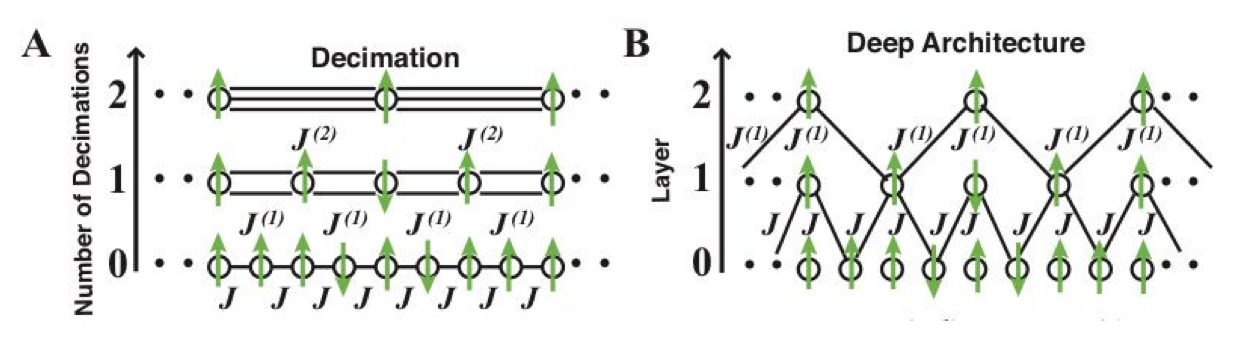From the 2014 paper by Mehta and Schwab where they introduced the map between RG and DNNs built by stacking RBMs. More details are provided in the remaining sections of the present article (source).

There are many other works in the literature connecting renormalization and deep learning, following different strategies and having distinct goals. In particular, the work of Naftali Tishby and collaborators based on the information bottleneck method is fascinating. Also, Mehta and Schwab explained the map for only one type of neural network, and subsequent work already exists. However, for conciseness, I will focus here on their original paper, since their insight was responsible for giving rise to a large volume of relevant subsequent work on the topic.

Before giving a relatively detailed description (see this article for a great, though much less technical, description) of this relationship I will provide some of the nitty-gritty of both RG theory of and RBMs.

### Renormalization Group Theory: A Bird’s-eye View

As mentioned above, renormalization involves the application of coarse-graining techniques to physical systems. RG theory is a general conceptual framework so one needs methods to operationalize those concepts. Variational Renormalization group (VRG) is one such scheme which was proposed by Kadanoff, Houghton and Yalabik in 1976.

For clarity of exposition, I chose to focus on one specific type of system to illustrate how RG works, namely, quantum spin systems, instead of proceeding in full generality. But before delving into the mathematical machinery, I will give a “hand waving” explanation of the meaning of spin in physics.

#### The Concept of Spin in Physics

In physics, spin can be defined as “an intrinsic form of angular momentum carried by elementary particles, composite particles, and atomic nuclei.” Though spin is by definition a quantum mechanical concept having no classical counterpart, particles with spin are often (though incorrectly) depicted as small tops rotating around their own axis. Spins are closely related to the phenomenon of magnetism.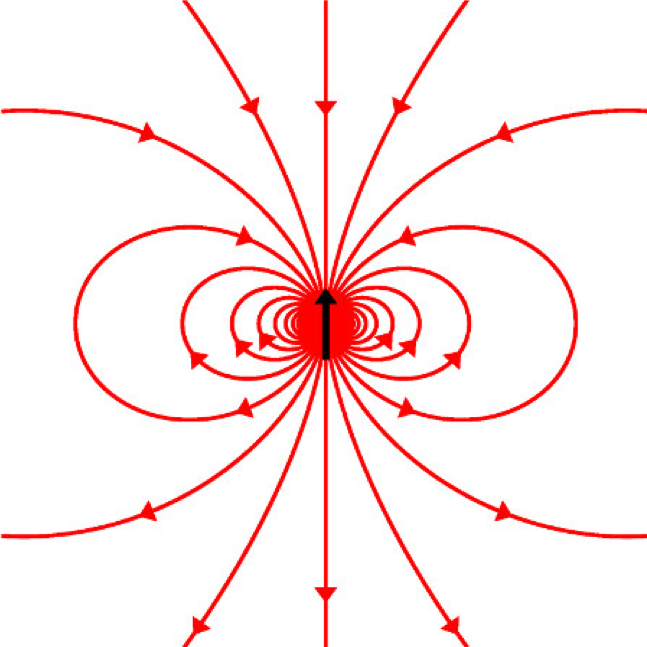The particle spin (black arrow) and its associated magnetic field lines (source).

#### The Mathematics of Renormalization

Let us consider a system or ensemble of N spins. For visualization purposes suppose they can be put on a lattice, as illustrated in the figure below.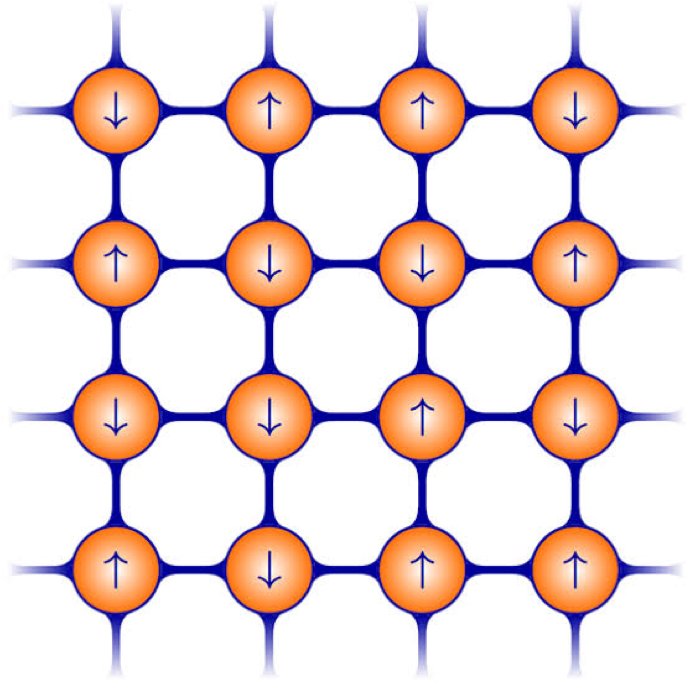A 2-dimensional lattice of spins (represented by the little arrows). The spheres are charged atoms (source).

Since spins can be up or down, they are associated with binary variablesThe index i can be used to label the position of the spin in the lattice. For convenience, I will represent a configuration of spins by a vector v.For systems in thermal equilibrium, the probability distribution associated with a spin configuration v has the following form:This is the ubiquitous Boltzmann distribution (with the temperature set to 1 for convenience). The object H(v) is the so-called Hamiltonian of the system, which can be defined as “an operator corresponding to the sum of the kinetic [and] potential energies for all the particles in the system”. The denominator Z is a normalization factor known as the partition functionThe Hamiltonian of the system can be expressed as a sum of terms corresponding to interactions between spins:The set of parametersare called coupling constants and they determine the strength of the interactions between spins (second term) or between spins and external magnetic fields (first term).

Another important quantity we will need to consider is the free energy. Free energy is a concept originally from thermodynamics where it is defined as “the energy in a physical system that can be converted to do work”. Mathematically, it is given in our case by:The symbol “tr” stands for trace (from linear algebra). In the present context, it represents the sum over all possible configurations of visible spins v.

At each step of the renormalization procedure, the behavior of the system at small length scales is averaged out. The Hamiltonian of the coarse-grained system is expressed in terms of new coupling constantsand new, coarse-grained variables are obtained. In our case, the latter are block spins h and the new Hamiltonian is:To better understand what are block spins, consider the two-dimensional lattice below. Each arrow represents a spin. Now divide the lattice into square blocks each containing 2×2 spins. The block spins are the average spins corresponding to each of these blocks.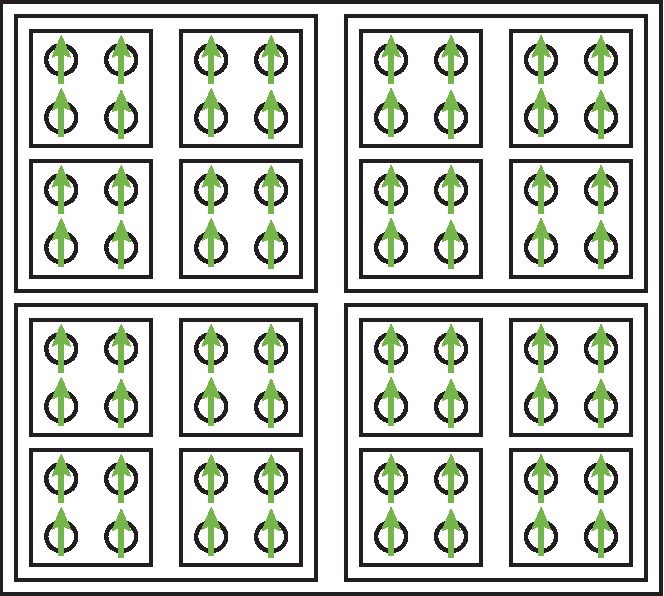In block spin RG, the system is coarse-grained into new block variables describing the effective behavior of spin blocks (source).

Note that the new Hamiltonian has the same structure as the original one, only with configurations of blocks of spins in place of physical spins.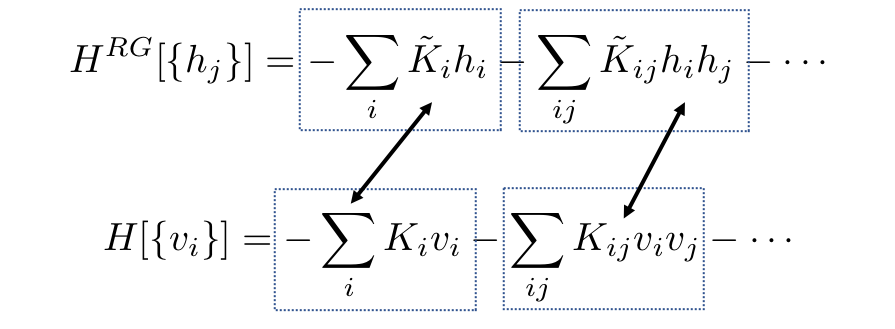Both Hamiltonians have the same structure but with different variables and couplings.

In other words, the form of the model does not change but as we zoom out the parameters of the model change. The full renormalization of the theory is obtained by systematically repeating these steps. After several RG iterations, some of the parameters will be dropped out and some will remain. The ones that remain are called relevant operators.

A connection between these Hamiltonians is obtained by the requirement that the free energy (described a few lines above) does not change after an RG-transformation.

#### Variational Renormalization group (VRG)

As mentioned above, to implement the RG mappings one can use the variational renormalization group (VRG) scheme. In this scheme, the mappings are implemented by an operatorwhere λ is a set of parameters. This operator encodes the couplings between hidden and input (visible) spins and satisfies the following relation:which defines the new Hamiltonian given above. Though in an exact RG transformation, the coarse-grained system would have exactly the same free energy as the original system i.e.which is equivalent to the following conditionin practice, this condition cannot be satisfied exactly and variational schemes are used to find λ that minimizes the difference between the free energiesor equivalently, to approximate the exact RG transformation.

### A Quick Summary of RBMs

I have described in some detail the internal workings of restricted Boltzmann machines in a previous article. Here I will provide a more condensed explanation.

Restricted Boltzmann Machines (RBMs) are generative, energy-based models. used for nonlinear unsupervised feature learning. Their simplest version consists of two layers only:

• One layer of visible units which will be denoted by v
• One hidden layer with units denoted by h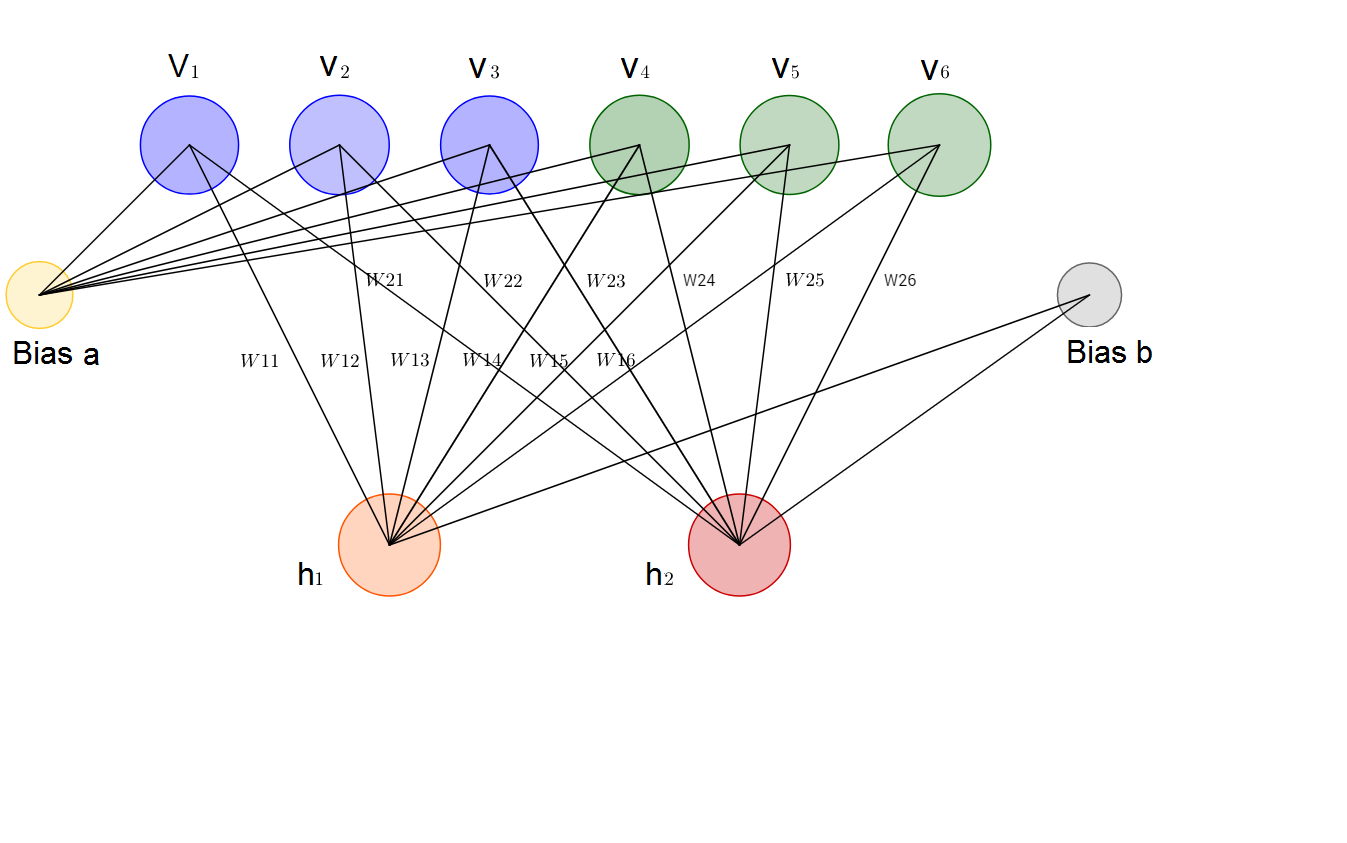Illustration of a simple Restricted Boltzmann Machine (source).

Again I will consider a binary visible dataset v with n elements extracted from some probability distributionEq. 9: Probability distribution of the input or visible data.

The hidden units in the RBM (represented by the vector h) are coupled to the visible units with interaction energy given byThe energy sub-index λ represents the set of variational parameters {c, b, W}. where the first two elements are vectors and the third one is a matrix. The goal of RBMs is to output a λ-dependent probability distribution that is as close as possible to the distribution of the input data P(v).

The probability associated with a configuration (v,h) and parameters λ is a function of this energy functional:From this joint probability, one can easily obtain the variational (marginalized) distribution of visible units by summing over the hidden units. Likewise, the marginalized distribution of hidden units is obtained by summing over the visible units:We can define an RBM Hamiltonian as follows:The λ parameters can be chosen to optimize the so-called Kullback-Leibler (KL) divergence or relative entropy which measures how different two probability distributions are. In the present case, we are interested in the KL divergence between the true data distribution and the variational distribution of the visible units produced by the RBM. More specifically:When both distributions are identical:### Exactly mapping RG and RBM

Mehta and Schwab showed that to establish the exact mapping between RG and RBMs, one can choose the following expression for the variational operator:Recall that the Hamiltonian H(v) contains encoded inside it the probability distribution of the input data. With this choice of variational operator, one can quickly prove the RG Hamiltonian and the RBM Hamiltonian on the hidden layer are the same:Also, when an exact RG transformation can be implemented, the true and variational Hamiltonian are identical:Hence we see that one step of the renormalization group with spins v and block-spins h can be exactly mapped into a two-layered RBM made of visible units v and hidden units h.

As we stack increasingly more layers of RBMs we are in effect performing more and more rounds of the RG transformation.

#### Application to the Ising Model

Following this rationale, we conclude that RBMs, a type of unsupervised deep learning algorithm, implements the variational RG process. This is a remarkable correspondence and Mehta and Schwab demonstrate their idea by implementing stacked RBMs on a well-understood Ising spin model. They fed, as input data, spin configurations sampled from an Ising model into the DNN. Their results show that, remarkably, DNNs seem to be performing (Kadanoff) block spin renormalization.

In the authors’ words “Surprisingly, this local block spin structure emerges from the training process, suggesting the DNN is self-organizing to implement block spin renormalization… I was astounding to us that you don’t put that in by hand, and it learns”.

Their results show that, remarkably, DNNs seem to be performing block spin renormalization.

In the figure below from their paper, A shows the architecture of the DNN. In B the learning parameters W are plotted to show the interaction between hidden and visible units. In D we see the gradual formation of block spins (the blob in the picture) as we move from along the layers of the DNN. In E the RBM reconstructions reproducing the macroscopic structure of three data samples are shown.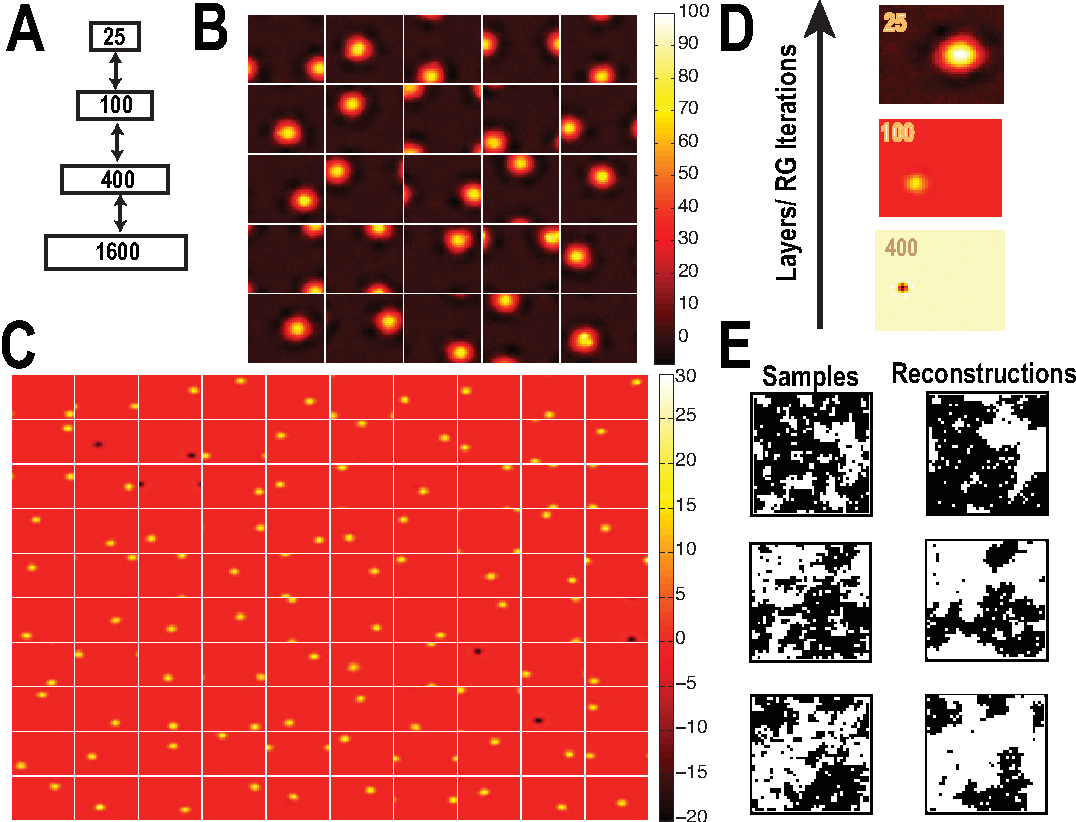Deep neural networks applied to the 2D Ising model. See the main text for a detailed description of each of the figures (source).

### Conclusions and Outlook

In 2014 it was shown by Mehta and Schwab that a Restricted Boltzmann Machine (RBM), a type of neural network, is connected to the renormalization group, a concept originally from physics. In the present article, I reviewed part of their analysis. As previously recognized, both RG and deep neural networks bear a remarkable “philosophical resemblance”: both distill complex systems into their relevant parts. This RG-RBM mapping is a kind of formalization of this similarity.

Since deep learning and biological learning processes have many similarities, it is not too much of a stretch to hypothesize that our brains may also use some kind of “renormalization on steroids” to make sense of our perceived reality.

As one of the authors suggested, “Maybe there is some universal logic to how you can pick out relevant features from data, I would say this is a hint that maybe something like that exists.”

It is not too much of a stretch to hypothesize that our brains may also use some kind of “renormalization on steroids” to make sense of our perceived reality.

The problem with this is that in contrast to self-similar systems (with fractal-like behavior) where RG works well, systems in nature generally are not self-similar. A possible way out of this limitation, as pointed out by the neuroscientist Terrence Sejnowski, would be if our brains somehow operated at critical points with all neurons influencing the whole network. But that is a topic for another article!

Thanks for reading and see you soon! As always, constructive criticism and feedback are always welcome!

My Github and personal website www.marcotavora.me have (hopefully) some other interesting stuff both about data science and about physics.

Views: 2141

Comment

Join Data Science Central